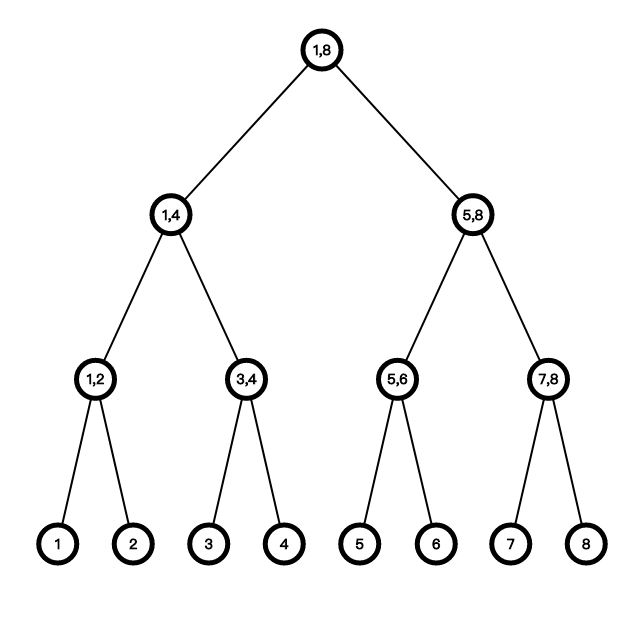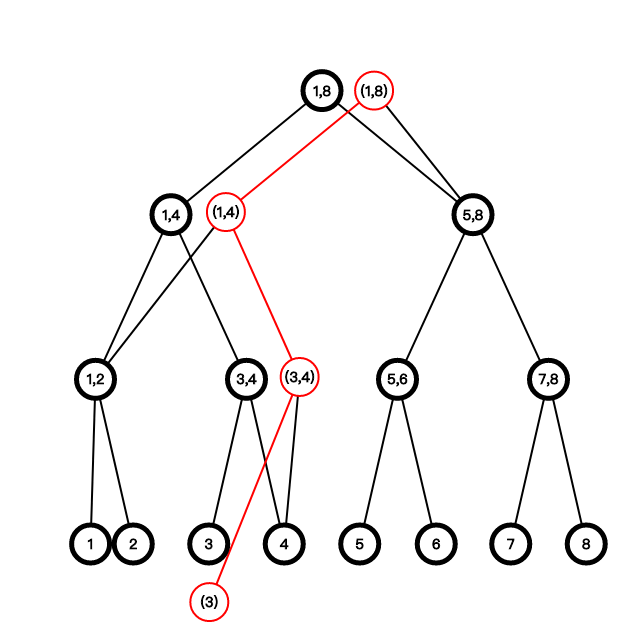# 简述

From Wikipedia.org

# 主席树的结构// Example on sustainable segment tree;

const int MAX_N = 1e5 + 2000;

struct node
{
int sum, lson, rson;
} nodes[MAX_N << 4];
// the entries for the history versions;
int roots[MAX_N];
// the amount of the nodes in the system;
int tot;
// generate the tree and return the new created node id.
int build(int l, int r)
{
int p = ++tot;
if (l == r)
return p;
int mid = (l + r) >> 1;
nodes[p].lson = build(l, mid), nodes[p].rson = build(mid + 1, r);
return p;
}
// given the previous version, to generate a new version since the update;
int update(int qx, int l, int r, int pre, int val)
{
int p = ++tot;
// copy the info and update;
nodes[p] = nodes[pre], nodes[p].sum += val;
if (l == r)
return p;
int mid = (l + r) >> 1;
// to edit son nodes;
if (qx <= mid)
nodes[p].lson = update(qx, l, mid, nodes[pre].lson, val);
else
nodes[p].rson = update(qx, mid + 1, r, nodes[pre].rson, val);
return p;
}

# 适用场景 A：第 k 小问题

int query(int l, int r, int previous, int now, int k)
{
int lsWeight = sum[nodes[now].lson] - sum[nodes[previous].lson];
if (l == r)
return l;
if (k <= lsWeight)
return query(l, mid, nodes[previous].lson, nodes[now].lson, k);
else
return query(mid + 1, r, nodes[previous].rson, nodes[now].rson, k - lsWeight);
}

# 适用场景 B：排列的区间交集

int query(int ql, int qr, int l, int r, int p)
{
if (ql <= l && r <= qr)
return nodes[p].sum;
int mid = (l + r) >> 1, ans = 0;
if (ql <= mid)
ans = query(ql, qr, l, mid, nodes[p].lson);
if (mid < qr)
ans += query(ql, qr, mid + 1, r, nodes[p].rson);
return ans;
}

int inquire(int l1, int r1, int l2, int r2)
{
return query(l1, r1, 1, n, roots[r2]) - query(l1, r1, 1, n, roots[l1 - 1]);
}

void build(int a[], int b[], int n, int m)
{
int pos[n];
for (int i = 1; i <= n; i++)
pos[a[i]] = i;
for (int i = 1; i <= m; i++)
roots[i] = update(pos[b[i]], 1, n, roots[i - 1], 1);
}

## 发布者## 《主席树总结》上有1条评论

1.aleph_blanc说道：

催更！（